# RD Sharma Solutions for Class 6 Maths Chapter 5: Negative Numbers and Integers Exercise 5.3

## RD Sharma Solutions for Class 6 Maths Exercise 5.3 PDF

BYJU’S has a set of subject matter experts who create exercise wise solutions after gaining better knowledge about the concepts. The solutions are answered in an accurate manner based on the weightage in the Class 6 exam. The students can gain a better hold on the concepts and master the subject with the help of RD Sharma Solutions. The free download of PDF is available which is a major source of reference for the students to ace the Class 6 exam. RD Sharma Solutions for Class 6 Maths Chapter 5 Negative Numbers and Integers Exercise 5.3 are provided here.

## RD Sharma Solutions for Class 6 Maths Chapter 5: Negative Numbers and Integers Exercise 5.3 Download PDF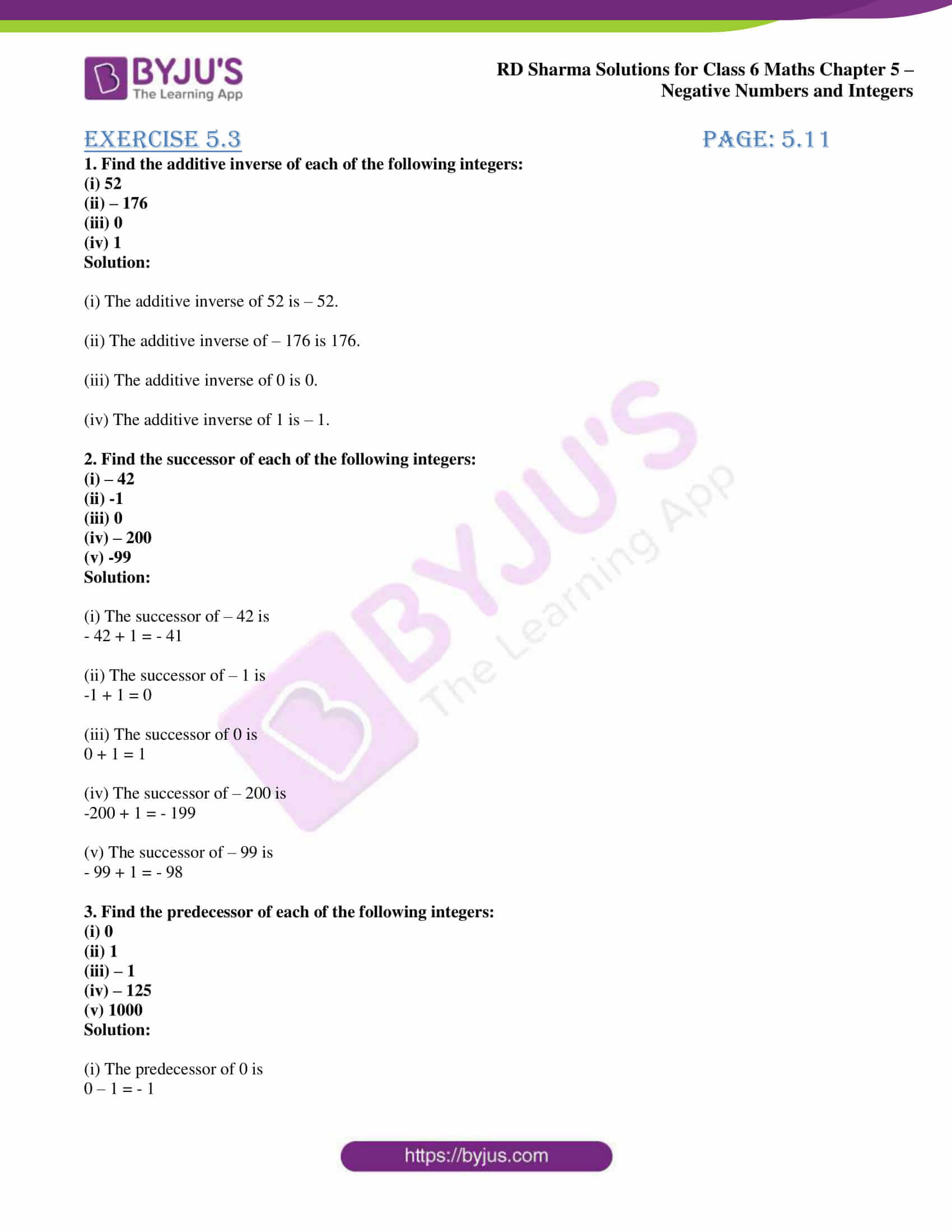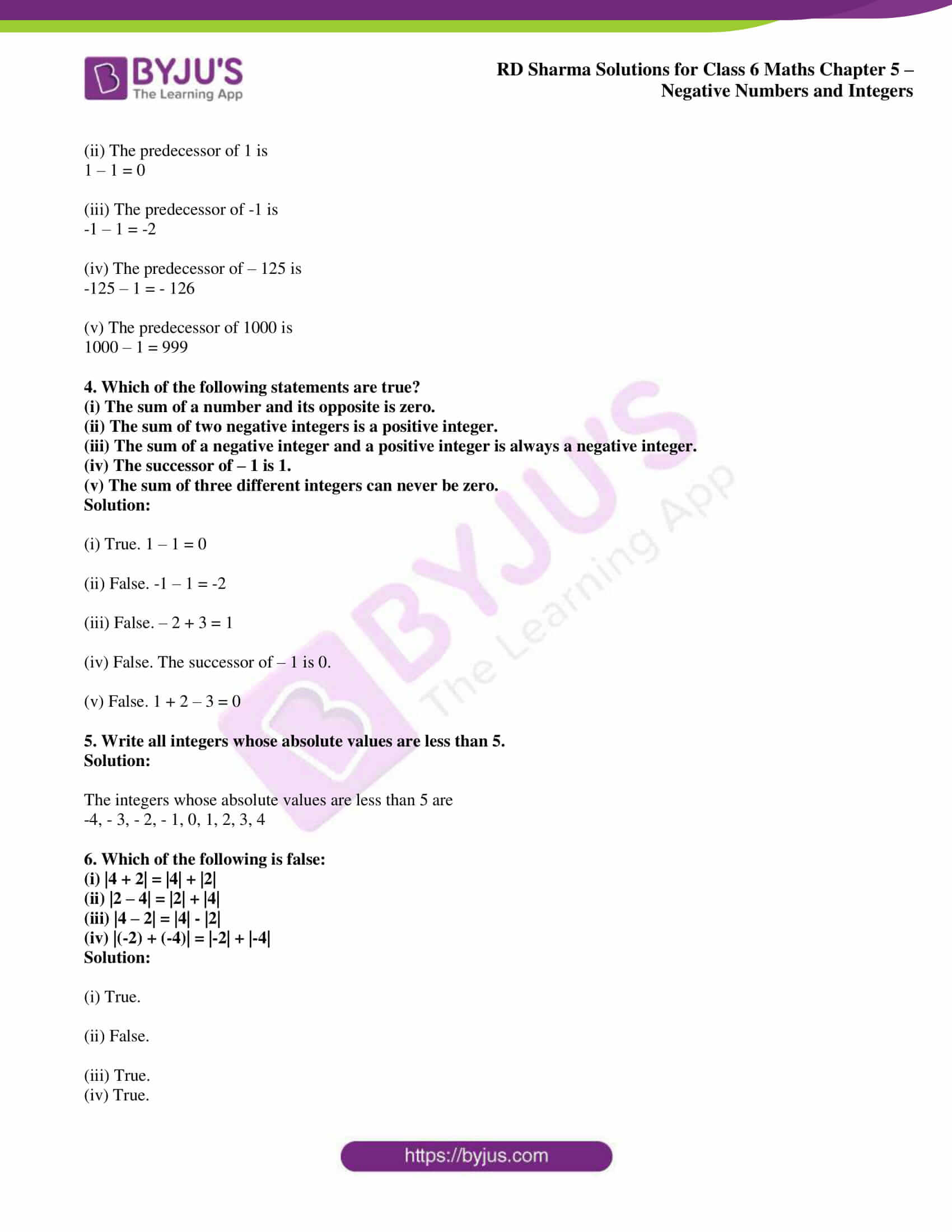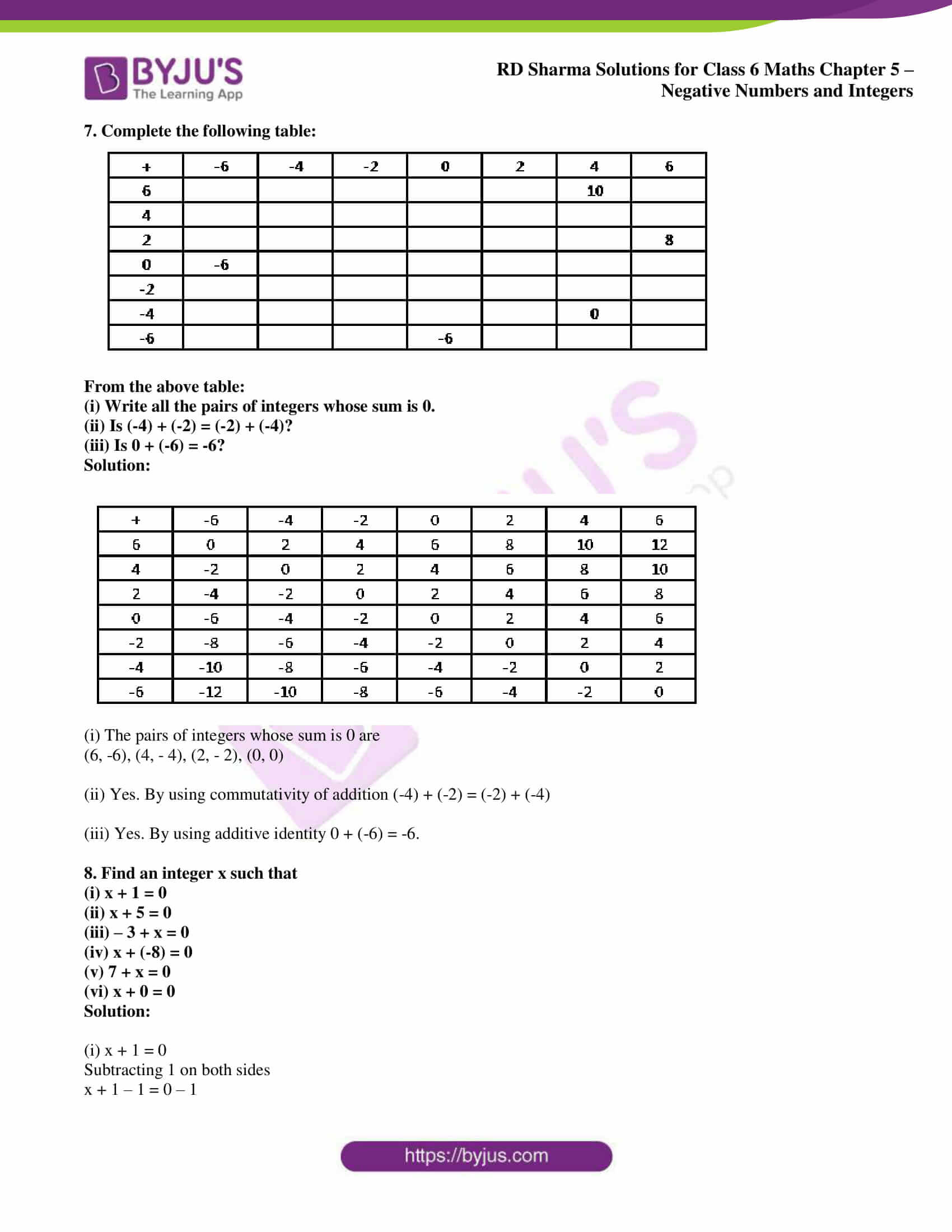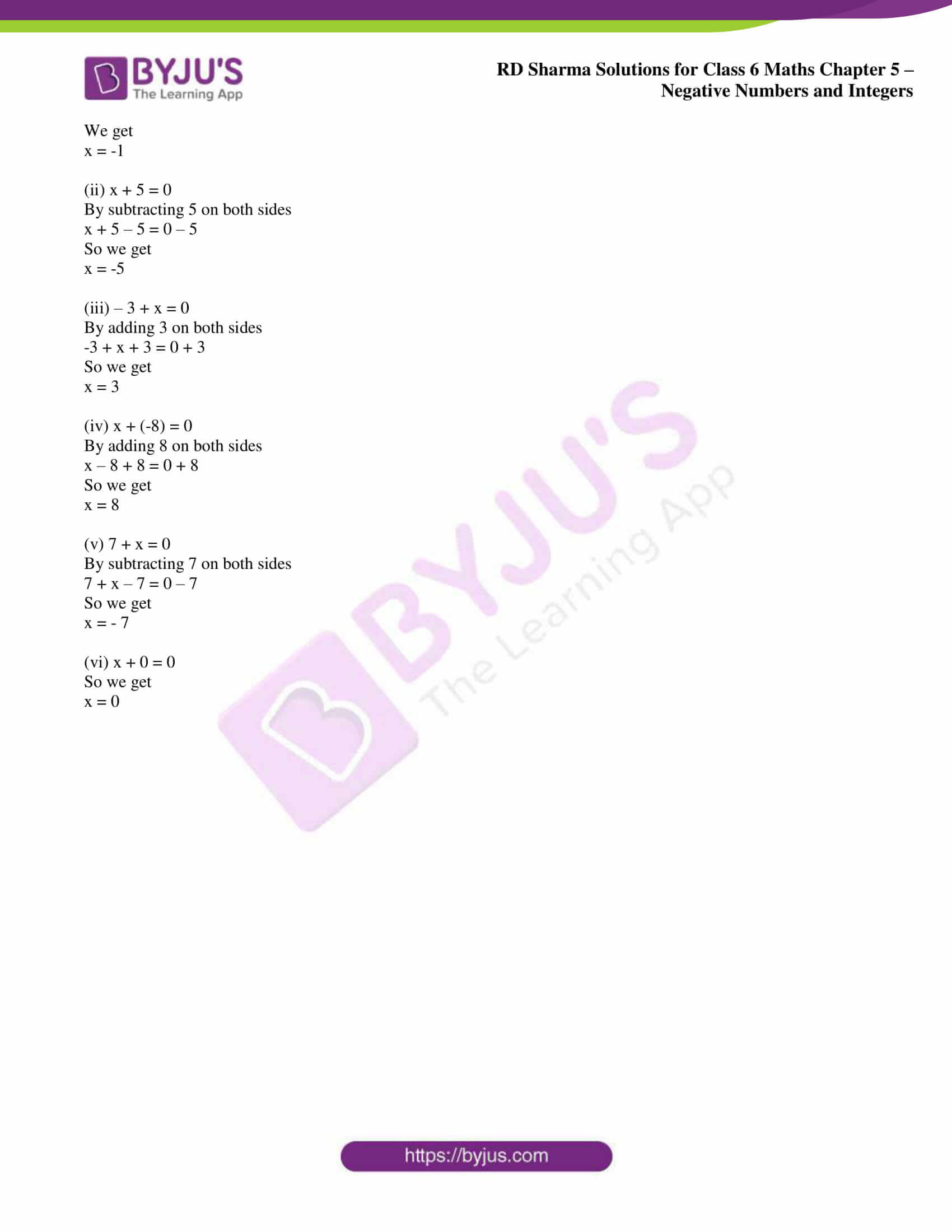## Access answers to Maths RD Sharma Solutions for Class 6 Chapter 5: Negative Numbers and Integers Exercise 5.3

1. Find the additive inverse of each of the following integers:

(i) 52

(ii) – 176

(iii) 0

(iv) 1

Solution:

(i) The additive inverse of 52 is – 52.

(ii) The additive inverse of – 176 is 176.

(iii) The additive inverse of 0 is 0.

(iv) The additive inverse of 1 is – 1.

2. Find the successor of each of the following integers:

(i) – 42

(ii) -1

(iii) 0

(iv) – 200

(v) -99

Solution:

(i) The successor of – 42 is

– 42 + 1 = – 41

(ii) The successor of – 1 is

-1 + 1 = 0

(iii) The successor of 0 is

0 + 1 = 1

(iv) The successor of – 200 is

-200 + 1 = – 199

(v) The successor of – 99 is

– 99 + 1 = – 98

3. Find the predecessor of each of the following integers:

(i) 0

(ii) 1

(iii) – 1

(iv) – 125

(v) 1000

Solution:

(i) The predecessor of 0 is

0 – 1 = – 1

(ii) The predecessor of 1 is

1 – 1 = 0

(iii) The predecessor of -1 is

-1 – 1 = -2

(iv) The predecessor of – 125 is

-125 – 1 = – 126

(v) The predecessor of 1000 is

1000 – 1 = 999

4. Which of the following statements are true?

(i) The sum of a number and its opposite is zero.

(ii) The sum of two negative integers is a positive integer.

(iii) The sum of a negative integer and a positive integer is always a negative integer.

(iv) The successor of – 1 is 1.

(v) The sum of three different integers can never be zero.

Solution:

(i) True. 1 – 1 = 0

(ii) False. -1 – 1 = -2

(iii) False. – 2 + 3 = 1

(iv) False. The successor of – 1 is 0.

(v) False. 1 + 2 – 3 = 0

5. Write all integers whose absolute values are less than 5.

Solution:

The integers whose absolute values are less than 5 are

-4, – 3, – 2, – 1, 0, 1, 2, 3, 4

6. Which of the following is false:

(i) |4 + 2| = |4| + |2|

(ii) |2 – 4| = |2| + |4|

(iii) |4 – 2| = |4| – |2|

(iv) |(-2) + (-4)| = |-2| + |-4|

Solution:

(i) True.

(ii) False.

(iii) True.

(iv) True.

7. Complete the following table: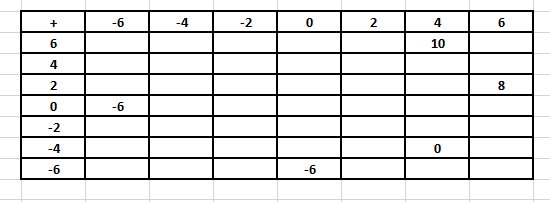From the above table:

(i) Write all the pairs of integers whose sum is 0.

(ii) Is (-4) + (-2) = (-2) + (-4)?

(iii) Is 0 + (-6) = -6?

Solution: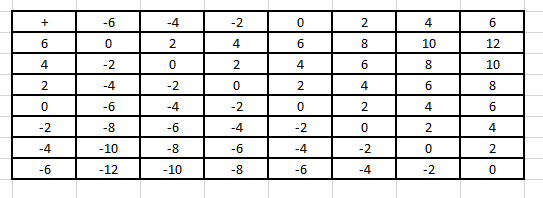(i) The pairs of integers whose sum is 0 are

(6, -6), (4, – 4), (2, – 2), (0, 0)

(ii) Yes. By using commutativity of addition (-4) + (-2) = (-2) + (-4)

(iii) Yes. By using additive identity 0 + (-6) = -6.

8. Find an integer x such that

(i) x + 1 = 0

(ii) x + 5 = 0

(iii) – 3 + x = 0

(iv) x + (-8) = 0

(v) 7 + x = 0

(vi) x + 0 = 0

Solution:

(i) x + 1 = 0

Subtracting 1 on both sides

x + 1 – 1 = 0 – 1

We get

x = -1

(ii) x + 5 = 0

By subtracting 5 on both sides

x + 5 – 5 = 0 – 5

So we get

x = -5

(iii) – 3 + x = 0

By adding 3 on both sides

-3 + x + 3 = 0 + 3

So we get

x = 3

(iv) x + (-8) = 0

By adding 8 on both sides

x – 8 + 8 = 0 + 8

So we get

x = 8

(v) 7 + x = 0

By subtracting 7 on both sides

7 + x – 7 = 0 – 7

So we get

x = – 7

(vi) x + 0 = 0

So we get

x = 0

### RD Sharma Solutions for Class 6 Maths Chapter 5 – Negative Numbers and Integers Exercise 5.3

RD Sharma Solutions Class 6 Maths Chapter 5 Negative Numbers and Integers Exercise 5.3 help students understand the properties of addition of integers and ways of solving problems using them.

### Key features of RD Sharma Solutions for Class 6 Maths Chapter 5: Negative Numbers and Integers Exercise 5.3

• The solutions created by the faculty at BYJU’S help students learn the important concepts more easily.
• Solving the exercise wise problems help students recognize the type of questions that would appear in the exam.
• Step wise solutions with explanations in a simple language help students understand concepts more thoroughly.
• Huge number of examples before each exercise contribute to students gaining a better practice of solving problems.